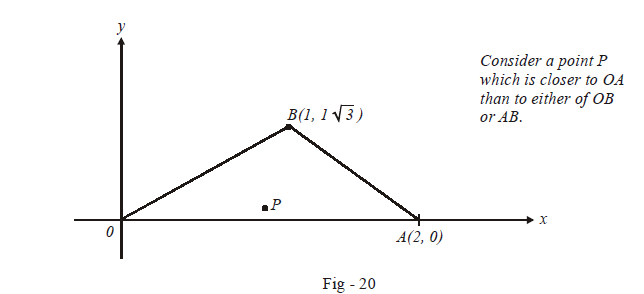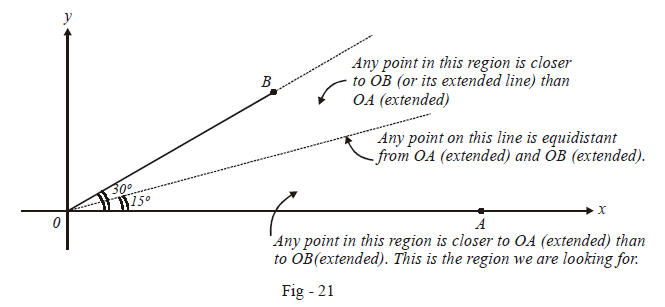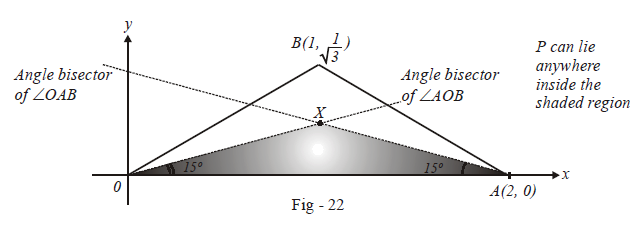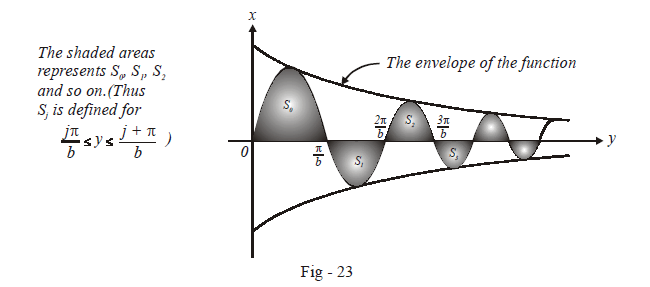# Problems On Areas Set-9

Go back to  'Areas and Volumes'

Example – 17

Let $$O (0,0), A(2,0)$$ and ($$B1,1/\sqrt 3$$) be the vertices of a triangle. Let $$R$$ be the region consisting of all those points $$P$$ inside $$\Delta OAB$$ which satisfy $$d(P,OA) {\rm{\le min}} \{ d(P,OB),d(P,AB)\} ,$$ where $$d$$ denotes the distance from the point to the corresponding line. Sketch the area $$R$$ and find its area.

Solution: Like the last question, this question too is more about correctly plotting the required region rather than calculating area. (In fact, we need not even use definite integration here since we’ll see that the region $$R$$ is bounded by straight lines).

Consider the triangle $$OAB$$ as described in the question:We need to find out the region in which $$P$$ can possibly lie.

Suppose that $$P$$ was equidistant from $$OA$$ and $$OB.$$ Then $$P$$ would lie on the angle bisector of $$\Delta AOB$$.

Since $$P$$ is actually closer to $$OA,$$ it lies ‘below’ the angle bisector, as shown in the next figure:Since $$P$$ also satisfies the constraint that it lies closer to $$OA$$ than to $$AB, P$$ will lie below the angle bisector of $$\Delta OAB$$ too. Thus, $$P$$ lies in the following regionIt is a matter of simple geometry now to evaluate the required area which is the area $$\Delta OAX$$ . Verify that this is $$\left( {\frac{{\sqrt 3 - 1}}{{\sqrt 3 + 1}}} \right)$$ sq. units.

Example – 18

Let $$b \ne 0$$ and for j = 0, 1, 2 ......, n, let $$S_j$$ be the area of the region bounded by the $$y-axis$$ and the curve $$x{e^{ax}} = \sin \,\, by$$.  Show that $${S_0},{S_1},{S_2}......,{S_n}$$ are in G.P.

Also, find their sum for $$a = {\rm{ }}-1\;{\rm{and}}\;{\rm{ }}b = \pi$$

Solution: The equation of the curve is

$x = {e^{ - ay}}\;\sin by$

If you recall our discussions on plotting graphs, you will realise that the graph (of x versus y) will be oscillatory in nature, bounded by an envelope given by $${e^{ - ay}}$$ . The zeroes of this function are given by.

\begin{align} by = j\pi \quad {\text{ }}j \in \mathbb{Z} \\ \Rightarrow \quad y = \frac{{j\pi }}{b} \quad{\text{ }}j \in \mathbb{Z} \\ \end{align}

Thus, we can now plot $$x$$ versus $$y$$ as follows:Now we proceed to calculate $${S_j}$$ :

\begin{align}{S_j}&= \left| {\int\limits_{j\pi /b}^{(j + 1)\pi /b} {{e^{ - ay}}\sin by\;{\rm{ }}dy} } \right| \\& = \left| {\left\{ {\frac{{{e^{ - ay}}}}{{{a^2} + {b^2}}}\left( { - a\sin by - b\cos by} \right)} \right\}} \right|_{\frac{{j\pi }}{b}}^{\frac{{(j + 1)\pi }}{b}}\left( \begin{array}{l}{\rm{we\;used\;integration\;by\;parts\;to\;evaluate\;}}\\{\rm{the \;indefinite\;integral;\;verify\;this\;step}}\end{array} \right)\\& = \left| {\left( {\frac{{{e^{ - \frac{a}{b}(j + 1)\pi }}}}{{{a^2} + {b^2}}} \times ( - b) \times {{( - 1)}^{j + 1}}} \right) - \left( {\frac{{{e^{ - \frac{a}{b}j\pi }}}}{{{a^2} + {b^2}}} \times ( - b) \times {{( - 1)}^j}} \right)} \right|\\& = \frac{{b{e^{ - ja\pi /b}}}}{{{a^2} + {b^2}}}\left( {1 + {e^{ - a\pi /b}}} \right) \qquad \qquad \qquad \qquad \qquad \qquad \qquad \dots (1)\end{align}

Thus,

$\frac{{{S_{j + 1}}}}{{{S_j}}} = {e^{ - a\pi /b}} \qquad which\; is \;a\; constant\\ \Rightarrow\quad {\rm{The\;series\;}}{{\rm{S}}_{\rm{0}}}{\rm{, }}{{\rm{S}}_{\rm{1}}}{\rm{, }}{{\rm{S}}_{\rm{2}}}{\rm{ }}......\;{{\rm{S}}_{\rm{n}}}\;{\rm{ is\;a \;G}}{\rm{.P}}{\rm{.}}$

For $$a = {\rm{ }} - 1\;and\;b = \pi ,$$ we have from (1):

\begin{align}&\qquad \qquad \; {s_j} = \frac{\pi }{{1 + {\pi ^2}}}{e^j}\left( {1 + e} \right)\\ &\Rightarrow \quad \sum\limits_{j = 0}^n {{s_j}}= \frac{{\pi (1 + e)}}{{{\pi ^2} + 1}}\left( {\frac{{{e^{n + 1}} - 1}}{{e - 1}}} \right) \\ \end{align}